CAT  >  Introduction: Angles & Lines

# Introduction: Angles & Lines - Notes | Study Quantitative Aptitude (Quant) - CAT

 Table of contentsAnglesProperties of Lines1 Crore+ students have signed up on EduRev. Have you?

Angles

Based on the measurement, angles have been classified into different groups.

1. Complementary Angles

• Two angles taken together are said to be complementary if the sum of measurement of the angles equal to 90o
• If ∠ A + ∠ B = 90o then ∠A is complementary of ∠B and vice versa.

2. Supplementary Angles

• Two angles are supplementary if the sum of their measure is 180o
• If ∠ A + ∠ B = 180o then ∠A is supplementary of ∠B and vice – versa.

Question for Introduction: Angles & Lines
Try yourself:Two angles whose sum is equal to 180° are called:

➢ Linear Pair

• Two angles were drawn on the same point and have one arm common. The sum of these angles is 180o, then they are said to be linear pair of angles.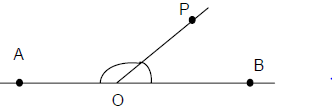• ∠AOP and ∠POB are linear pair of angles.

• Two angles are adjacent if and only if they have one common arm between them.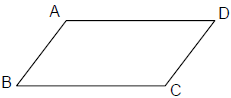• In the above figure, ∠ABC and ∠BCD are adjacent angles, since they have BC as their common arm.

Properties of Lines

• A line consists of infinite dots. A line is drawn by joining any two different points on a plane. Two different lines drawn can be either parallel or intersecting depending on their nature.
• If two lines intersect at a point, then they form two pairs of opposite angles (as shown in the figure), which are known as vertically opposite angles and have same measure. In the figure, ∠PRQ and ∠SRT are vertically opposite angles.
• Also, ∠QRS and ∠PRT are vertically opposite angles.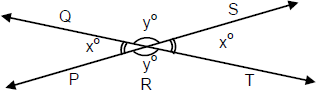• Also, ∠x + ∠y = 180o and are Linear pair angles.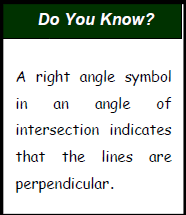➢ Perpendicular Lines

• An angle that has a measure of 90o is a right angle. If two lines intersect at right angles, the lines are perpendicular.
Example: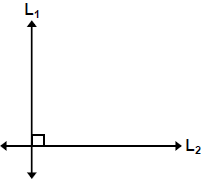L1 and L2 above are perpendicular and denoted by L1 ⊥ L2.

➢ Parallel Lines

• Two lines drawn on a plane are said to be parallel if they do not intersect each other. In the figure below lines, L1 and L2 are parallel and denoted by L1 || L2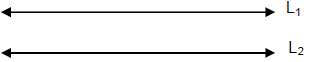➢ Parallel lines and Transverse

• If a common line intersects two parallel lines L1 and L2, then that common line is known as transverse.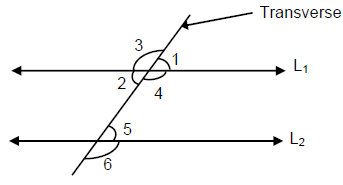• Pair of corresponding angles = (∠1 & ∠5) and (∠4 & ∠ 6)
• Pair of internal alternate angles = (∠2 & ∠5)
• Pair of exterior alternate angles = (∠3 & ∠6)
• Vertically opposite angles = ∠3 & ∠4
• For parallel lines intersected by the transversal, the pair of corresponding angles, interior alternate angles and exterior alternate angles are equal.
• ∠1 = ∠5, ∠2 = ∠5, ∠3 = ∠6 and ∠3 = ∠4

Question for Introduction: Angles & Lines
Try yourself:If AB || CD, EF ⊥ CD and ∠GED = 135° as per the figure given below. The value of ∠AGE is: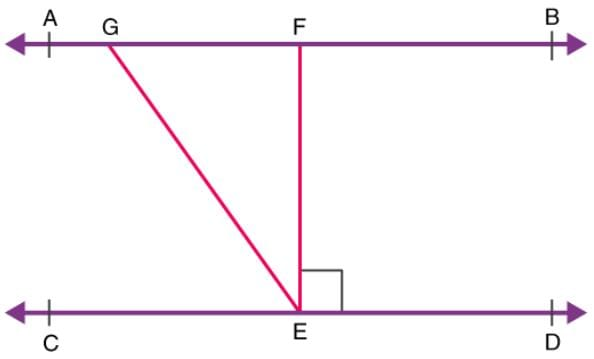The document Introduction: Angles & Lines - Notes | Study Quantitative Aptitude (Quant) - CAT is a part of the CAT Course Quantitative Aptitude (Quant).
All you need of CAT at this link: CAT

## Quantitative Aptitude (Quant)

163 videos|163 docs|131 tests
 Use Code STAYHOME200 and get INR 200 additional OFF

## Quantitative Aptitude (Quant)

163 videos|163 docs|131 tests

### How to Prepare for CAT

Read our guide to prepare for CAT which is created by Toppers & the best Teachers

Track your progress, build streaks, highlight & save important lessons and more!

,

,

,

,

,

,

,

,

,

,

,

,

,

,

,

,

,

,

,

,

,

;